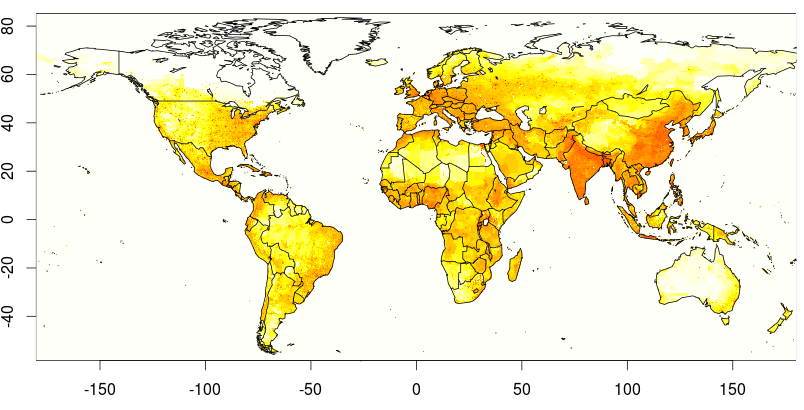Want to share your content on R-bloggers? click here if you have a blog, or here if you don't.

A few months ago, I did mention that France was a country with strong inequalities, especially when you look at higher education, and research teams. Paris has almost 50% of the CNRS researchers, while only 3% of the population lives there.

It looks like Paris is the only city, in France. And I wanted to check that, indeed, France is a country with strong inequalities, when we look at population density.

Using data from sedac.ciesin.columbia.edu, it is possible to get population density on a small granularity level,

```> rm(list=ls())
+      "/home/charpentier/glp00ag.asc",
+      skip=6)
> X=t(as.matrix(base,ncol=8640))
> X=X[,ncol(X):1]```

The scales for latitudes and longitudes can be obtained from the text file,

```> #ncols         8640
> #nrows         3432
> #xllcorner     -180
> #yllcorner     -58
> #cellsize      0.0416666666667```

Hence, we have

```> library(maps)
> world=map(database="world")
> vx=seq(-180,180,length=nrow(X)+1)
> vx=(vx[2:length(vx)]+vx[1:(length(vx)-1)])/2
> vy=seq(-58,85,length=ncol(X)+1)
> vy=(vy[2:length(vy)]+vy[1:(length(vy)-1)])/2```

If we plot our density, as in a previous post, on Where People Live,

```> I=seq(1,nrow(X),by=10)
> J=seq(1,ncol(X),by=10)
> image(vx[I],vy[J],log(1+X[I,J]),
+ col=rev(heat.colors(101)))
> lines(world[],world[])```we can see that we have a match, between the big population matrix, and polygons of countries.

Consider France, for instance. We can download the contour polygon with higher precision,

```> library(rgdal)
+ "fr.rds")
> n=length([email protected][]@Polygons)
> L=rep(NA,n)
> for(i in 1:n) L[i]=nrow([email protected][]@Polygons[[i]]@coords)
> idx=which.max(L)
> polygon_Fr=
+       [email protected][]@Polygons[[idx]]@coords
> min_poly=apply(polygon_Fr,2,min)
> max_poly=apply(polygon_Fr,2,max)
> idx_i=which((vx>min_poly)&(vx idx_j=which((vy>min_poly)&(vy sub_X=X[idx_i,idx_j]
> image(vx[idx_i],vy[idx_j],
+       log(sub_X+1),col=rev(heat.colors(101)),
+       xlab="",ylab="")
> lines(polygon_Fr)```We are now able to extract information about population for France, only (actually, it is only mainland France, islands are not considered here… to avoid complicated computations

```> library(sp)
> xy=expand.grid(x = vx[idx_i], y = vy[idx_j])
> dim(xy)
 65730     2```

Here, we have 65,730 small squares, in France.

```> pip=point.in.polygon(xy[,1],xy[,2],
+     polygon_Fr[,1],polygon_Fr[,2])>0
> dim(pip)=dim(sub_X)
> Fr=sub_X[pip]
> sum(Fr)
 58105272```

Observe that the total population within the French polygon is close to 60 million people, which is consistent with actual figures. Now, if we look more carefully at repartition over the French territory

```> library(ineq)
> Gini(Fr)
 0.7296936```

Gini coefficient is rather high (over 70%), but it is also possible to visualize Lorenz curve,

`> plot(Lc(Fr))`Observe that in 5% of the territory, we can find almost 54% of the population

```> 1-min(LcF\$L[LcF\$p>.95])
 0.5462632```

In order to compare with other countries, consider the

```> LC=function(rds="fr.rds"){
+ n=length([email protected][]@Polygons)
+ L=rep(NA,n)
+ for(i in 1:n)
L[i]=nrow([email protected][]@Polygons[[i]]@coords)
+ idx=which.max(L)
+ polygon_Fr=
+      [email protected][]@Polygons[[idx]]@coords
+ min_poly=apply(polygon_Fr,2,min)
+ max_poly=apply(polygon_Fr,2,max)
+ idx_i=which((vx>min_poly)&(vxmin_poly)&(vy0
+ dim(pip)=dim(sub_X)
+ Fr=sub_X[pip]
+ return(list(gini=Gini(Fr),LC=Lc(Fr))
+ }
> FRA=LC()```

For instance, consider Germany, or Italy

```> deu=download.file(
> DEU=LC("deu.rds")
> ITA=LC("ita.rds")```

It is possible to plot Lorenz curve, together,

```> plot(FRA\$LC,col="blue")
> lines(DEU\$LC,col="black")
> lines(ITA\$LC,col="red")```Observe that France is clearly below the other ones. Compared with Germany, there is a significant difference

```> FRA\$gini
 0.7296936
> DEU\$gini
 0.5088853```

More precisely, if 54% of French people live in 5% of the territory, only 40% of Italians, and 32% of the Germans,

```> 1-min(FRA\$LC\$L[FRA\$LC\$p>.95])
 0.5462632
> 1-min(ITA\$LC\$L[ITA\$LC\$p>.95])
 0.3933227
> 1-min(DEU\$LC\$L[DEU\$LC\$p>.95])
 0.3261124```